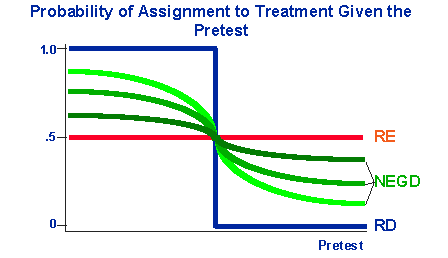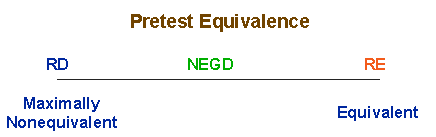Pre-Post Design Relationships

# Relationships Among Pre-Post DesignsThere are three major types of pre-post program-comparison group designs all sharing the basic design structure shown in the notation above:

The designs differ in the method by which participants are assigned to the two groups. In the RE, participants are assigned randomly. In the RD design, they are assigned using a cutoff score on the pretest. In the NEGD, assignment of participants is not explicitly controlled – they may self select into either group, or other unknown or unspecified factors may determine assignment.

Because these three designs differ so critically in their assignment strategy, they are often considered distinct or unrelated. But it is useful to look at them as forming a continuum, both in terms of assignment and in terms of their strength with respect to internal validity.

We can look at the similarity three designs in terms of their assignment by graphing their assignment functions with respect to the pretest variable. In the figure, the vertical axis is the probability that a specific unit (e.g., person) will be assigned to the treatment group). These values, because they are probabilities, range from 0 to 1. The horizontal axis is an idealized pretest score.Let’s first examine the assignment function for the simple pre-post randomized experiment. Because units are assigned randomly, we know that the probability that a unit will be assigned to the treatment group is always 12 or .5 (assuming equal assignment probabilities are used). This function is indicated by the horizontal red line at .5 in the figure. For the RD design, we arbitrarily set the cutoff value at the midpoint of the pretest variable and assume that we assign units scoring below that value to the treatment and those scoring at or above that value to the control condition (the arguments made here would generalize to the case of high-scoring treatment cases as well). In this case, the assignment function is a simple step function, with the probability of assignment to the treatment = 1 for the pretest scores below the cutoff and = 0 for those above. It is important to note that for both the RE and RD designs it is an easy matter to plot their assignment functions because assignment is explicitly controlled. This is not the case for the NEGD. Here, the idealized assignment function differs depending on the degree to which the groups are nonequivalent on the pretest. If they are extremely nonequivalent (with the treatment group scoring lower on the pretest), the assignment function would approach the step function of the RD design. If the groups are hardly nonequivalent at all, the function would approach the flat-line function of the randomized experiment.

The graph of assignment functions points an important issue about the relationships among these designs – the designs are not distinct with respect to their assignment functions, they form a continuum. On one end of the continuum is the RE design and at the other is the RD. The NEGD can be viewed as a degraded RD or RE depending on whether the assignment function more closely approximates one or the other.

We can also view the designs on a continuum with respect to the degree to which they generate a pretest difference between the groups.The figure shows that the RD design induces the maximum possible pretest difference. The RE design induces the smallest pretest difference (the most equivalent). The NEGD fills in the gap between these two extreme cases. If the groups are very nonequivalent, the design is closer to the RD design. If they’re very similar, it’s closer to the RE design.

Finally, we can also distinguish the three designs in terms of the a priori knowledge they give about assignment. It should be clear that in the RE design we know perfectly the probability of assignment to treatment – it is .5 for each participant. Similarly, with the RD design we also know perfectly the probability of assignment. In this case it is precisely dependent on the cutoff assignment rule. It is dependent on the pretest where the RE design is not. In both these designs, we know the assignment function perfectly, and it is this knowledge that enables us to obtain unbiased estimates of the treatment effect with these designs. This is why we conclude that, with respect to internal validity, the RD design is as strong as the RE design. With the NEGD however, we do not know the assignment function perfectly. Because of this, we need to model this function either directly or indirectly (e.g., through reliability corrections).

The major point is that we should not look at these three designs as entirely distinct. They are related by the nature of their assignment functions and the degree of pretest nonequivalence between groups. This continuum has important implications for understanding the statistical analyses of these designs.